Corresponding Angles homework Help at TutorEye

# Best Homework Help For Corresponding Angles

## Top Questions

t schedule 3 people for the day my % scheduled is 78.57% My question is how did the computer come up with the 78.57%?
View More

I would love to get a written solution. I did the other proof tree that you can see in the attaached files. So its supposed to look like that Thank You.
View More

xtbook. i really need in in half an hour
View More

## Corresponding Angles:

The angles generated when two parallel lines are crossed by the transversal are known as corresponding angles.

## Corresponding Angles Sample Questions:

Question 1: Find the value of x°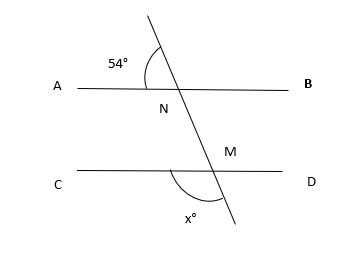(a) 120°

(b) 126°

(c) 110°

(d) 100°

Explanation: Sum of linear pair angle is 180°

Question 2: Find the angle x°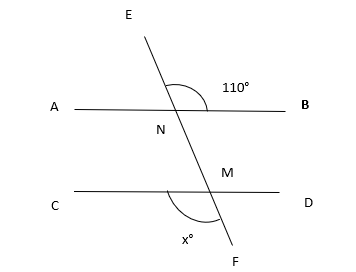(a) 120°

(b) 126°

(c) 110°

(d) 100°

Explanation: Use the vertical opposite and corresponding angle rule.

Question 3: Find the value of x°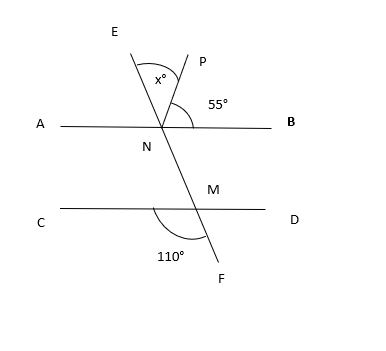(a) 50°

(b) 56°

(c) 55°

(d) 100°

Explanation: Use the vertical opposite and corresponding angle rule.

Question 4: Find the value of x°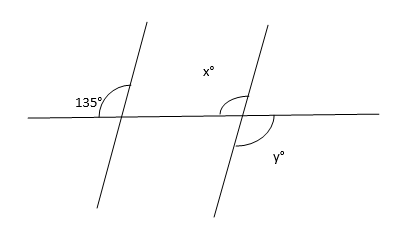(a) 135°

(b) 55°

(c) 130°

(d) 65°

Explanation: Use the pair of correspomding angle rule.

Question 5: Find the value of y°(a) 55°

(b) 135°

(c) 120°

(d) 75°

Explanation: Use the vertically opposite angle rule.

Question 6: Find the value of x°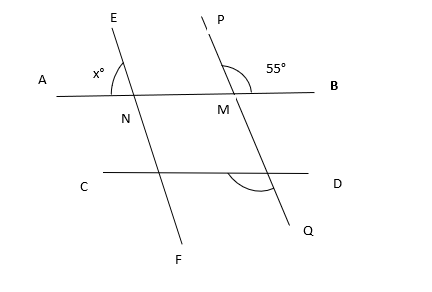(a) 135°

(b) 125°

(c) 115°

(d) 105°

Explanation: Sum of linear pair angle is 180°

Pair of corresponding angles are equal

Question 7: Angle C and R are which angle?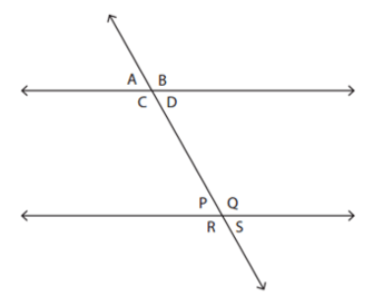(a) Alternate interior angles

(b) Vertically opposite angles

(c) Consecutive interior angles

(d) Corresponding interior angles

Explanation: Angle C and R are pairs of corresponding interior angles.

Question 8: Angles on the same side of a transversal, in corresponding positions, and are congruent are called _____.

(a) Alternate angles

(b) Corresponding angles

(c) Vertically opposite angle

(d) None

Explanation: Angles on the same side of a transversal, in corresponding positions, and are congruent are called  Corresponding angles

Question 9: In the below figure;

AE||BD, ∠CED = 54°,∠EFC = 105°

Find ∠FCE?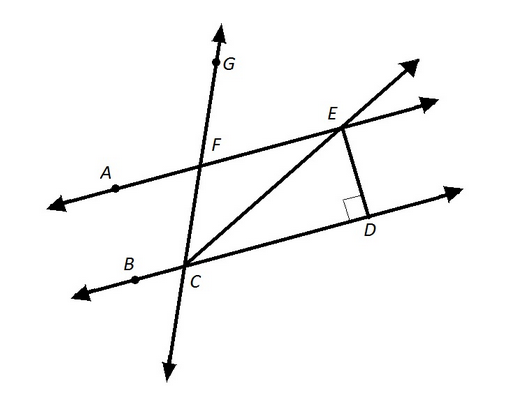(a) 54°

(b) 34°

(c) 39°

(d) 36°

Explanation: Alternate interior angles are equal

Sum of linear pair angle is 180°

Question 10: Find the  value of x°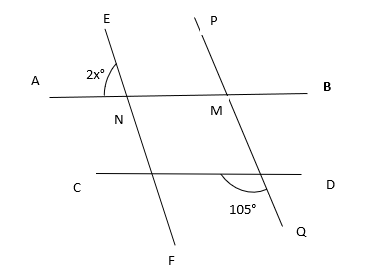(a) 37.5°

(b) 37°

(c) 36.5°

(d) 36°